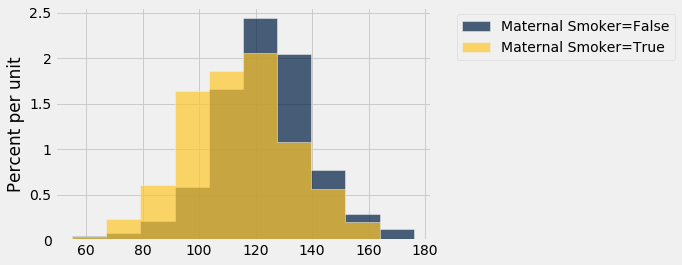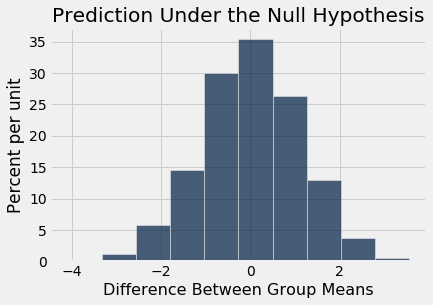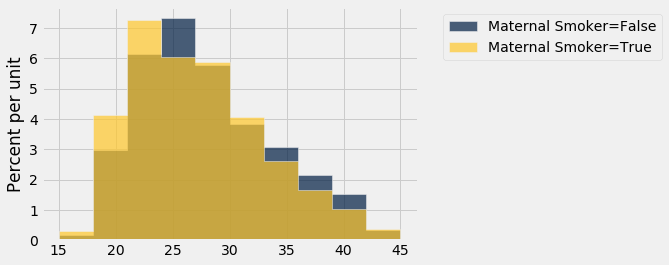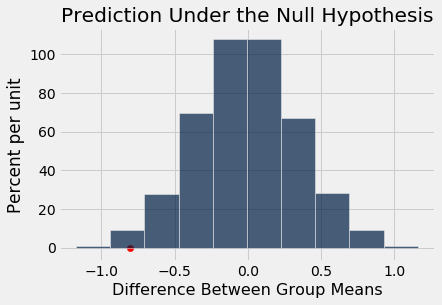In :
from datascience import *
path_data = '../../../../data/'
import numpy as np

import matplotlib
matplotlib.use('Agg', warn=False)
%matplotlib inline
import matplotlib.pyplot as plots
plots.style.use('fivethirtyeight')


### A/B Testing¶

In modern data analytics, deciding whether two numerical samples come from the same underlying distribution is called A/B testing. The name refers to the labels of the two samples, A and B.

We will develop the method in the context of an example. The data come from a sample of newborns in a large hospital system. We will treat it as if it were a simple random sample though the sampling was done in multiple stages. Stat Labs by Deborah Nolan and Terry Speed has details about a larger dataset from which this set is drawn.

### Smokers and Nonsmokers¶

The table births contains the following variables for 1,174 mother-baby pairs: the baby's birth weight in ounces, the number of gestational days, the mother's age in completed years, the mother's height in inches, pregnancy weight in pounds, and whether or not the mother smoked during pregnancy.

In :
births = Table.read_table(path_data + 'baby.csv')
births

Out:
Birth Weight Gestational Days Maternal Age Maternal Height Maternal Pregnancy Weight Maternal Smoker
120 284 27 62 100 False
113 282 33 64 135 False
128 279 28 64 115 True
108 282 23 67 125 True
136 286 25 62 93 False
138 244 33 62 178 False
132 245 23 65 140 False
120 289 25 62 125 False
143 299 30 66 136 True
140 351 27 68 120 False

... (1164 rows omitted)

One of the aims of the study was to see whether maternal smoking was associated with birth weight. Let's see what we can say about the two variables.

We'll start by selecting just Birth Weight and Maternal Smoker. There are 715 non-smokers among the women in the sample, and 459 smokers.

In :
smoking_and_birthweight = births.select('Maternal Smoker', 'Birth Weight')

In :
smoking_and_birthweight.group('Maternal Smoker')

Out:
Maternal Smoker count
False 715
True 459

Let's look at the distribution of the birth weights of the babies of the non-smoking mothers compared to those of the smoking mothers. To generate two overlaid histograms, we will use hist with the optional group argument which is a column label or index. The rows of the table are first grouped by this column and then a histogram is drawn for each one.

In :
smoking_and_birthweight.hist('Birth Weight', group = 'Maternal Smoker')The distribution of the weights of the babies born to mothers who smoked appears to be based slightly to the left of the distribution corresponding to non-smoking mothers. The weights of the babies of the mothers who smoked seem lower on average than the weights of the babies of the non-smokers.

This raises the question of whether the difference reflects just chance variation or a difference in the distributions in the larger population. Could it be that there is no difference between the two distributions in the population, but we are seeing a difference in the samples just because of the mothers who happened to be selected?

### The Hypotheses¶

We can try to answer this question by a test of hypotheses. The chance model that we will test says that there is no underlying difference in the popuations; the distributions in the samples are different just due to chance.

Formally, this is the null hypothesis. We are going to have to figure out how to simulate a useful statistic under this hypothesis. But as a start, let's just state the two natural hypotheses.

Null hypothesis: In the population, the distribution of birth weights of babies is the same for mothers who don't smoke as for mothers who do. The difference in the sample is due to chance.

Alternative hypothesis: In the population, the babies of the mothers who smoke have a lower birth weight, on average, than the babies of the non-smokers.

### Test Statistic¶

The alternative hypothesis compares the average birth weights of the two groups and says that the average for the mothers who smoke is smaller. Therefore it is reasonable for us to use the difference between the two group means as our statistic.

We will do the subtraction in the order "average weight of the smoking group $-$ average weight of the non-smoking group". Small values (that is, large negative values) of this statistic will favor the alternative hypothesis.

The observed value of the test statistic is about $-9.27$ ounces.

In :
means_table = smoking_and_birthweight.group('Maternal Smoker', np.average)
means_table

Out:
Maternal Smoker Birth Weight average
False 123.085
True 113.819
In :
means = means_table.column(1)
observed_difference = means.item(1) - means.item(0)
observed_difference

Out:
-9.266142572024918

We are going compute such differences repeatedly in our simulations below, so we will define a function to do the job. The function takes three arguments:

• the name of the table of data
• the label of the column that contains the numerical variable whose average is of interest
• the label of the column that contains the Boolean variable for grouping

It returns the difference between the means of the True group and the False group.

In :
def difference_of_means(table, label, group_label):
reduced = table.select(label, group_label)
means_table = reduced.group(group_label, np.average)
means = means_table.column(1)
return means.item(1) - means.item(0)


To check that the function is working, let's use it to calculate the observed difference between the means of the two groups in the sample.

In :
difference_of_means(births, 'Birth Weight', 'Maternal Smoker')

Out:
-9.266142572024918

That's the same as the value of observed_difference calculated earlier.

### Predicting the Statistic Under the Null Hypothesis¶

To see how the statistic should vary under the null hypothesis, we have to figure out how to simulate the statistic under that hypothesis. A clever method based on random permutations does just that.

If there were no difference between the two distributions in the underlying population, then whether a birth weight has the label True or False with respect to maternal smoking should make no difference to the average. The idea, then, is to shuffle all the labels randomly among the mothers. This is called random permutation.

Take the difference of the two new group means: the mean weight of the babies whose mothers have been randomly labeled smokers and the mean weight of the babies of the remaining mothers who have all been randomly labeled non-smokers. This is a simulated value of the test statistic under the null hypothesis.

Let's see how to do this. It's always a good idea to start with the data.

In :
smoking_and_birthweight

Out:
Maternal Smoker Birth Weight
False 120
False 113
True 128
True 108
False 136
False 138
False 132
False 120
True 143
False 140

... (1164 rows omitted)

There are 1,174 rows in the table. To shuffle all the labels, we will draw a random sample of 1,174 rows without replacement. Then the sample will include all the rows of the table, in random order.

We can use the Table method sample with the optional with_replacement=False argument. We don't have to specify a sample size, because by default, sample draws as many times as there are rows in the table.

In :
shuffled_labels = smoking_and_birthweight.sample(with_replacement = False).column(0)
original_and_shuffled = smoking_and_birthweight.with_column('Shuffled Label', shuffled_labels)

In :
original_and_shuffled

Out:
Maternal Smoker Birth Weight Shuffled Label
False 120 False
False 113 False
True 128 True
True 108 False
False 136 True
False 138 True
False 132 True
False 120 False
True 143 True
False 140 True

... (1164 rows omitted)

Each baby's mother now has a random smoker/non-smoker label in the column Shuffled Label, while her original label is in Maternal Smoker. If the null hypothesis is true, all the random re-arrangements of the labels should be equally likely.

Let's see how different the average weights are in the two randomly labeled groups.

In :
shuffled_only = original_and_shuffled.drop('Maternal Smoker')
shuffled_group_means = shuffled_only.group('Shuffled Label', np.average)
shuffled_group_means

Out:
Shuffled Label Birth Weight average
False 119.709
True 119.078

The averages of the two randomly selected groups are quite a bit closer than the averages of the two original groups. We can use our function difference_of_means to find the two differences.

In :
difference_of_means(original_and_shuffled, 'Birth Weight', 'Shuffled Label')

Out:
-0.6306595365418843
In :
difference_of_means(original_and_shuffled, 'Birth Weight', 'Maternal Smoker')

Out:
-9.266142572024918

But could a different shuffle have resulted in a larger difference between the group averages? To get a sense of the variability, we must simulate the difference many times.

As always, we will start by defining a function that simulates one value of the test statistic under the null hypothesis. This is just a matter of collecting the code that we wrote above. But because we will later want to use the same process for comparing means of other variables, we will define a function that takes three arguments:

• the name of the table of data
• the label of the column that contains the numerical variable
• the label of the column that contains the Boolean variable for grouping

It returns the difference between the means of two groups formed by randomly shuffling all the labels.

In :
def one_simulated_difference(table, label, group_label):
shuffled_labels = table.sample(with_replacement = False
).column(group_label)
shuffled_table = table.select(label).with_column(
'Shuffled Label', shuffled_labels)
return difference_of_means(shuffled_table, label, 'Shuffled Label')


Run the cell below a few times to see how the output changes.

In :
one_simulated_difference(births, 'Birth Weight', 'Maternal Smoker')

Out:
-1.8218839983545791

### Permutation Test¶

Tests based on random permutations of the data are called permutation tests. We are performing one in this example. In the cell below, we will simulate our test statistic – the difference between the averages of the two groups – many times and collect the differences in an array.

In :
differences = make_array()

repetitions = 5000
for i in np.arange(repetitions):
new_difference = one_simulated_difference(births, 'Birth Weight', 'Maternal Smoker')
differences = np.append(differences, new_difference)


The array differences contains 5,000 simulated values of our test statistic: the difference between the mean weight in the smoking group and the mean weight in the non-smoking group, when the labels have been assigned at random.

### Conclusion of the Test¶

The histogram below shows the distribution of these 5,000 values. It is the empirical distribution of the test statistic simulated under the null hypothesis. This is a prediction about the test statistic, based on the null hypothesis.

In :
Table().with_column('Difference Between Group Means', differences).hist()
print('Observed Difference:', observed_difference)
plots.title('Prediction Under the Null Hypothesis');

Observed Difference: -9.266142572024918Notice how the distribution is centered around 0. This makes sense, because under the null hypothesis the two groups should have roughly the same average. Therefore the difference between the group averages should be around 0.

The observed difference in the original sample is about $-9.27$ ounces, which doesn't even appear on the horizontal scale of the histogram. The observed value of the statistic and the predicted behavior of the statistic under the null hypothesis are inconsistent.

The conclusion of the test is that the data favor the alternative over the null. The average birth weight of babies born to mothers who smoke is less than the average birth weight of babies born to non-smokers.

If you want to compute an empirical P-value, remember that low values of the statistic favor the alternative hypothesis.

In :
empirical_P = np.count_nonzero(differences <= observed_difference) / repetitions
empirical_P

Out:
0.0

The empirical P-value is 0, meaning that none of the 5,000 permuted samples resulted in a difference of -9.27 or lower. This is only an approximation. The exact chance of getting a difference in that range is not 0 but it is vanishingly small.

### Another Permutation Test¶

We can use the same method to compare other attributes of the smokers and the non-smokers, such as their ages. Histograms of the ages of the two groups show that in the sample, the mothers who smoked tended to be younger.

In :
smoking_and_age = births.select('Maternal Smoker', 'Maternal Age')
smoking_and_age.hist('Maternal Age', group = 'Maternal Smoker')The observed difference between the average ages is about $-0.8$ years.

In :
observed_age_difference = difference_of_means(births, 'Maternal Age', 'Maternal Smoker')
observed_age_difference

Out:
-0.8076725017901509

Remember that the difference is calculated as the mean age of the smokers minus the mean age of the non-smokers. The negative sign shows that the smokers are younger on average.

Is this difference due to chance, or does it reflect an underlying difference in the population?

As before, we can use a permutation test to answer this question. If the underlying distributions of ages in the two groups are the same, then the empirical distribution of the difference based on permuted samples will predict how the statistic should vary due to chance.

In :
age_differences = make_array()

repetitions = 5000
for i in np.arange(repetitions):
new_difference = one_simulated_difference(births, 'Maternal Age', 'Maternal Smoker')
age_differences = np.append(age_differences, new_difference)


The observed difference is in the tail of the empirical distribution of the differences simulated under the null hypothesis.

In :
Table().with_column('Difference Between Group Means', age_differences).hist()
plots.scatter(observed_age_difference, 0, color='red', s=40)
plots.title('Prediction Under the Null Hypothesis')
print('Observed Difference:', observed_age_difference)

Observed Difference: -0.8076725017901509The empirical P-value of the test is the proportion of simulated differences that were equal to or less than the observed difference. This is because low values of the difference favor the alternative hypothesis that the smokers were younger on average.

In :
empirical_P = np.count_nonzero(age_differences <= observed_age_difference) / 5000
empirical_P

Out:
0.0104

The empirical P-value is around 1% and therefore the result is statistically significant. The test supports the hypothesis that the smokers were younger on average.

In [ ]: